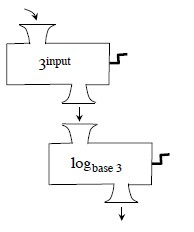### Home > PC > Chapter 3 > Lesson 3.2.2 > Problem3-76

3-76.

Joe has hooked up two function machines as shown in the diagram. When he puts $4$ into the “$3^{\text{input}}$” machine, $81$ comes out. Then the $81$ goes into the “$\log_{\text{base}3}$” machine.1. What comes out of the “$\log_{\text{base}3}$” machine when $81$ goes in?

$\log_381=4$ because $3^4=81$.

2. Now put $2$ into the “$3^{\text{input}}$” machine. What number comes out of this machine and goes into the “$\log_{\text{base}3}$” machine? What then comes out of the “$\log_{\text{base}3}$” machine?

$3^{2} = 9$.

$9$ goes into the $\log_3$ machine.

$\log_39=2$ because $3^{2} = 9$.

3. If $x$ goes into the “$3^{\text{input}}$” machine, $y$ comes out. If $y$ then goes into the “$\log_{\text{base}3}$” machine, what comes out? If $y$ then, …

$x$

4. If $x$ goes into the “$\log_{\text{base}3}$” machine, $y$ comes out. Write this in mathematical notation.

$\log_3x=y$

5. If this same $y$ goes into the “$3^{\text{input}}$” machine, the original $x$ comes out. Write this in mathematical notation.

$x = 3^{y}$

6. Do your answers to parts (d) and (e) say exactly the same thing?

Yes